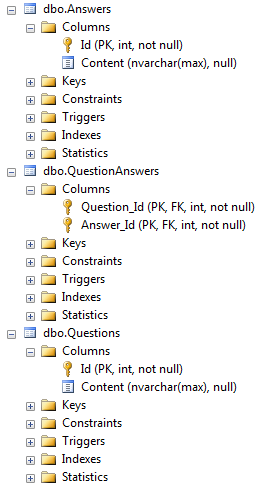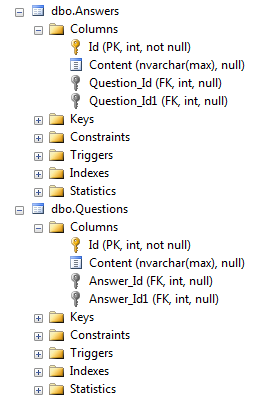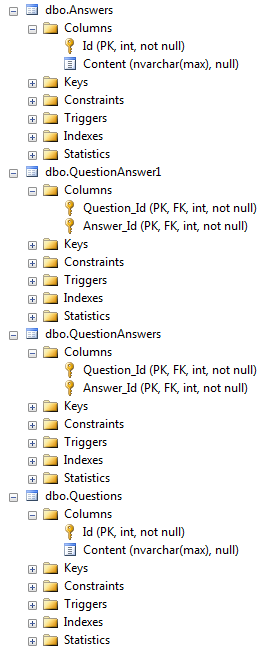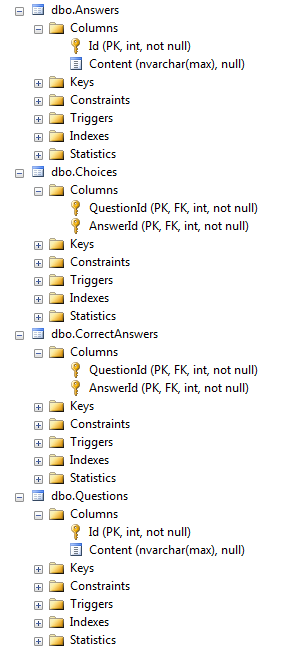OJ Develops

Thoughts on software development. .NET | C# | Azure

# Multiple Many-to-Many Associations - Entity Framework Code First

28 January 2014Entity Framework Code First lets you build domain classes first without an existing database. As soon as a database is needed, Entity Framework will create the database for you based on the shape of your classes. Entity Framework is smart enough to figure out things such as column names, column types, one-to-many associations, many-to-many associations, and more, according to your intentions. But sometimes, we have to explicitly tell the framework what our intentions are. In this post, we will talk about multiple many-to-many relationships.

## Code First Convention for Many-to-Many Relationships

How does Entity Framework know that there is a many-to-many relationship between two classes? By convention, if the two classes have collections of one another’s type, Entity Framework infers that there is a many-to-many relationship between those classes and thus creates the appropriate database tables.

For example, take a look at the following two classes:

``````public class Question
{
public int Id { get; set; }
public string Content { get; set; }

public IList<Answer> Choices { get; set; }
}

{
public int Id { get; set; }
public string Content { get; set; }

public IList<Question> QuestionsFor { get; set; }
}
``````

A question has multiple choices, and an answer can be a choice for many different questions. Both of these classes have collection properties of each other, and Entity Framework correctly infers that there is a many-to-many relationship between them. Following is a snapshot of the database structure created by Entity Framework:Aside from creating the Questions and Answers table, Entity Framework also created a join table named QuestionAnswers. In addition, it set all the necessary primary and foreign key relationships that tie those three tables together.

## Multiple Many-to-Many Associations

Now suppose we modify the classes in the following manner:

``````public class Question
{
public int Id { get; set; }
public string Content { get; set; }

public IList<Answer> Choices { get; set; }
}

{
public int Id { get; set; }
public string Content { get; set; }

public IList<Question> QuestionsFor { get; set; }
public IList<Question> CorrectAnswersFor { get; set; }
}
``````

Each question now has an additional list of correct answers, as a question can have more than one correct answer. Also, an answer can be the correct answer for more than one question, so a corresponding list of questions has also been added.

In the database, the intended result is to have an additional join table which would match the correct question-answer pairs.

However, when the database gets recreated, we get this:This is not at all what we wanted!

Note: if you try to do this with an existing database, you will get an error. To solve this, you can manually recreate the database, or use an appropriate initializer such as DropCreateDatabaseAlways.

## Configuration for Many-to-Many Relationships

This is the case when we have to explicitly tell Entity Framework what our intentions are. I am going to show how to solve this using Data Annotations and Fluent Configuration.

### Using Data Annotations

Let’s change the Question class to the following:

``````public class Question
{
public int Id { get; set; }
public string Content { get; set; }

[InverseProperty("QuestionsFor")]
public IList<Answer> Choices { get; set; }

}
``````

Also, we need to add a reference to the namespace:

``````using System.ComponentModel.DataAnnotations.Schema;
``````

The data annotations tell Entity Framework what properties in the Answer class these lists are supposed to be linked to. The argument of each annotation is the matching property name in the Answer class. You can place these annotations in the Question class (like we did), in the Answer class, or in both.

If we recreate the database, the structure would now look like this:This is the correct table structure. However, because of the default table naming conventions, we don’t know which join table contains the choices and which contains the correct answers. There’s no way to configure this using Data Annotations, so we are going to use Fluent Configuration instead.

### Using Fluent Configuration

To use Fluent Configuration, we can override the OnModelCreating method of DbContext. Let’s remove the data annotations and do this in our context class:

``````protected override void OnModelCreating(DbModelBuilder modelBuilder)
{
modelBuilder.Entity<Question>()
.HasMany(q => q.Choices)
.WithMany(q => q.QuestionsFor)
.Map(q =>
{
q.ToTable("Choices");
q.MapLeftKey("QuestionId");
});

modelBuilder.Entity<Question>()
.Map(q =>
{
q.MapLeftKey("QuestionId");